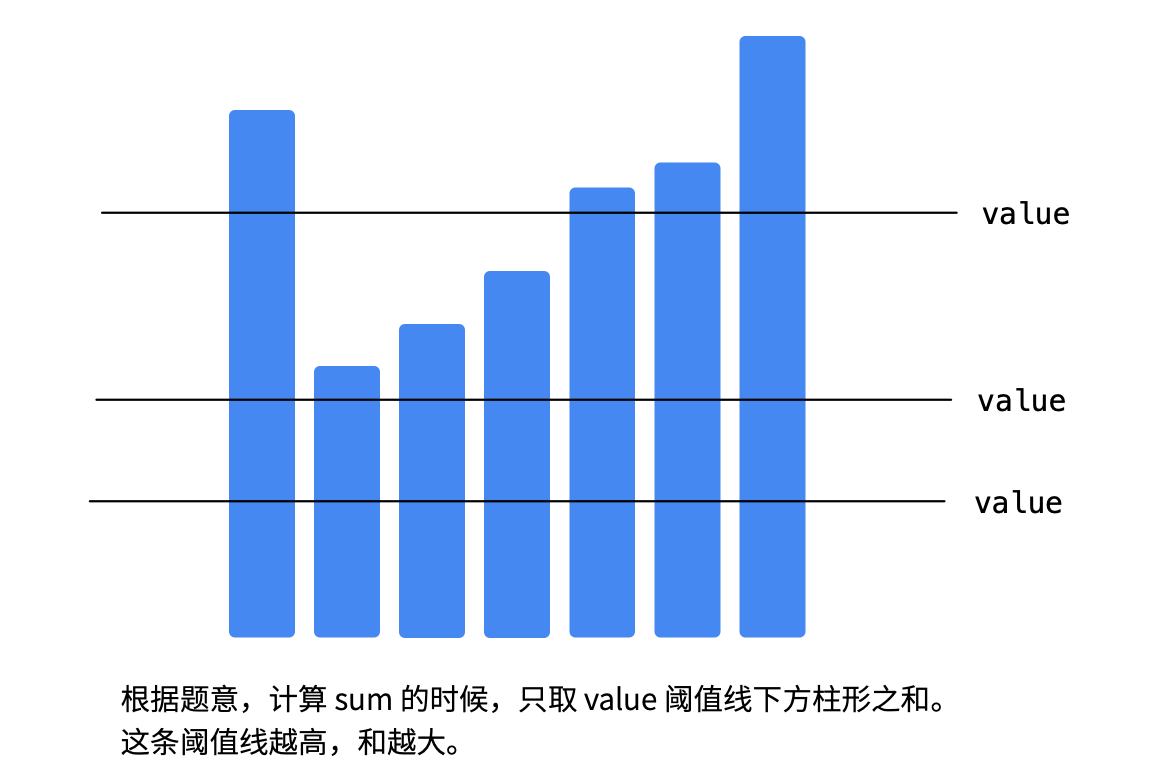# 1、转变数组后最接近目标值的数组和

输入：arr = [4,9,3], target = 10



输入：arr = [2,3,5], target = 10



输入：arr = [60864,25176,27249,21296,20204], target = 56803



## 1.1、解题思路## 1.2、算法

  /**
* 如果选择一个阈值 value ，使得它对应的 sum 是第 1 个大于等于 target 的，那么目标值可能在 value 也可能在 value - 1。
*/
public int findBestValue(int[] arr, int target) {
int left = 0;
int right = 0;
// 因为不能选超过数组中最大的数，选择一个最大的数right
for (int num : arr) {
right = Math.max(right, num);
}

// 找到一个最接近
while (left < right) {
int mid = (right + left) / 2;
int sum = calculateSum(arr, mid);
// 计算第 1 个使得转变后数组的和大于等于 target 的阈值 threshold
if (sum < target) {
// 严格小于的一定不是解
left = mid + 1;
} else {
right = mid;
}
}

// 比较阈值线分别定在 left - 1 和 left 的时候与 target 的接近程度
//left 是第一个大于 target的，所以 left-1一定不大于target
int sum1 = calculateSum(arr, left);
int sum2 = calculateSum(arr, left - 1);
if (target - sum2  <= sum1 - target ) {
return left - 1;
}
return left;
}

/**
* 计算在threshold 之下的面积
*/
private int calculateSum(int[] arr, int threshold) {
int sum = 0;
for (int num : arr) {
sum += Math.min(num, threshold);
}
return sum;
}


## 1.3、测试

  @Test
public void test() {
int[] arr = {2, 9, 2};
int target = 3;
System.out.println(findBestValue(arr, target));
}